# Selina Solutions Concise Maths Class 10 Chapter 13 Section and Mid-Point Formula

For any two given points in a Cartesian plane, the knowledge of co-ordinate geometry is essential to find: (i) the distance between the given points, (ii) the co-ordinates of a point which divides the line joining the given points in a given ratio and (iii) the co-ordinates of the mid-point of the line segment joining the two given points. Students in this chapter will solve problems on the section formula, points of trisection, mid-point formula and centroid of a triangle. The Selina Solutions for Class 10 Mathematics is the right resource for students to learn the correct methods of solving problems and also to clarify their doubts. All the solutions are prepared by subject matter experts at BYJU’S, according to the latest ICSE patterns. Further, students can access the Selina Solutions for Class 10 Mathematics Chapter 13 Section and Mid-Point Formula free PDF of all exercises in the links provided below.

## Selina Solutions Concise Maths Class 10 Chapter 13 Section and Mid-Point Formula Download PDF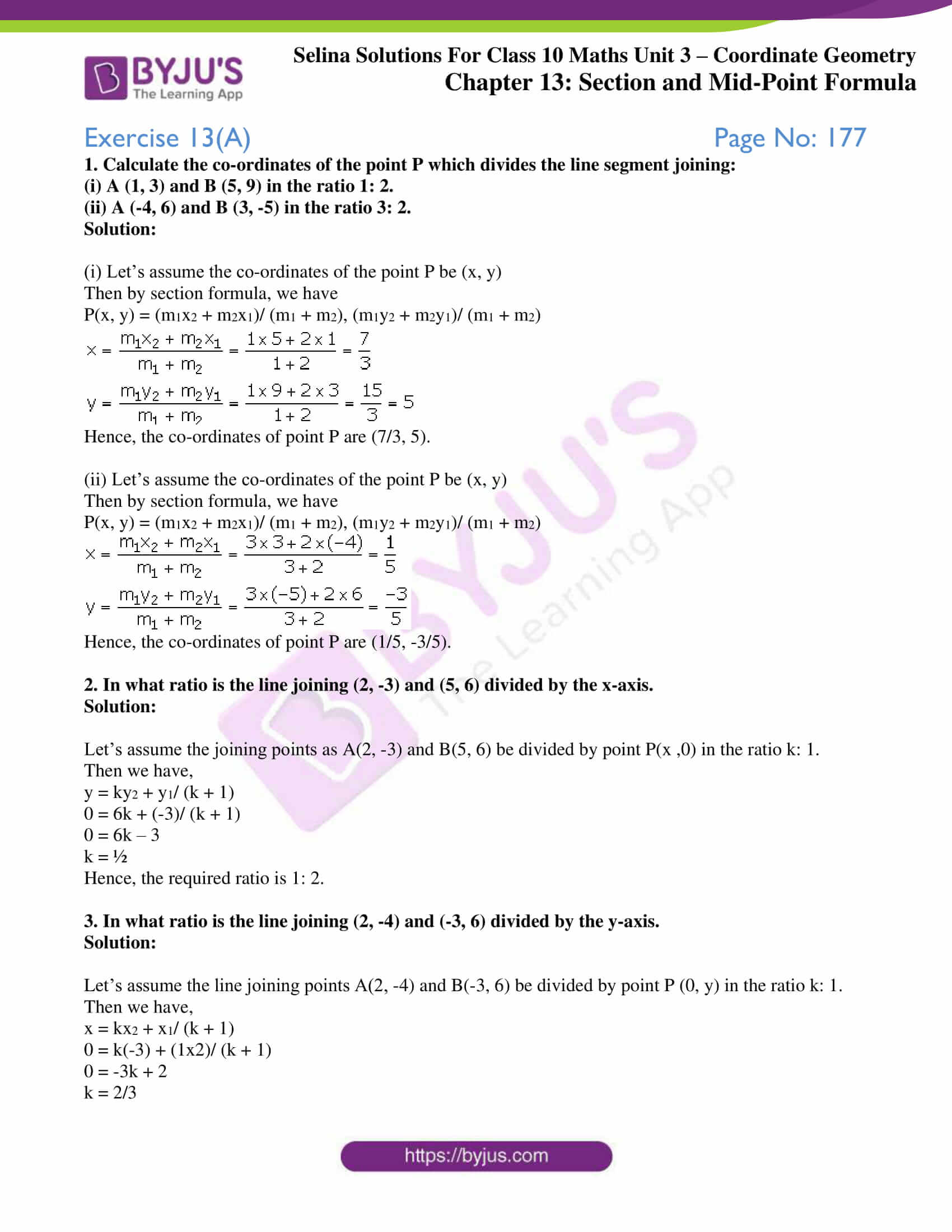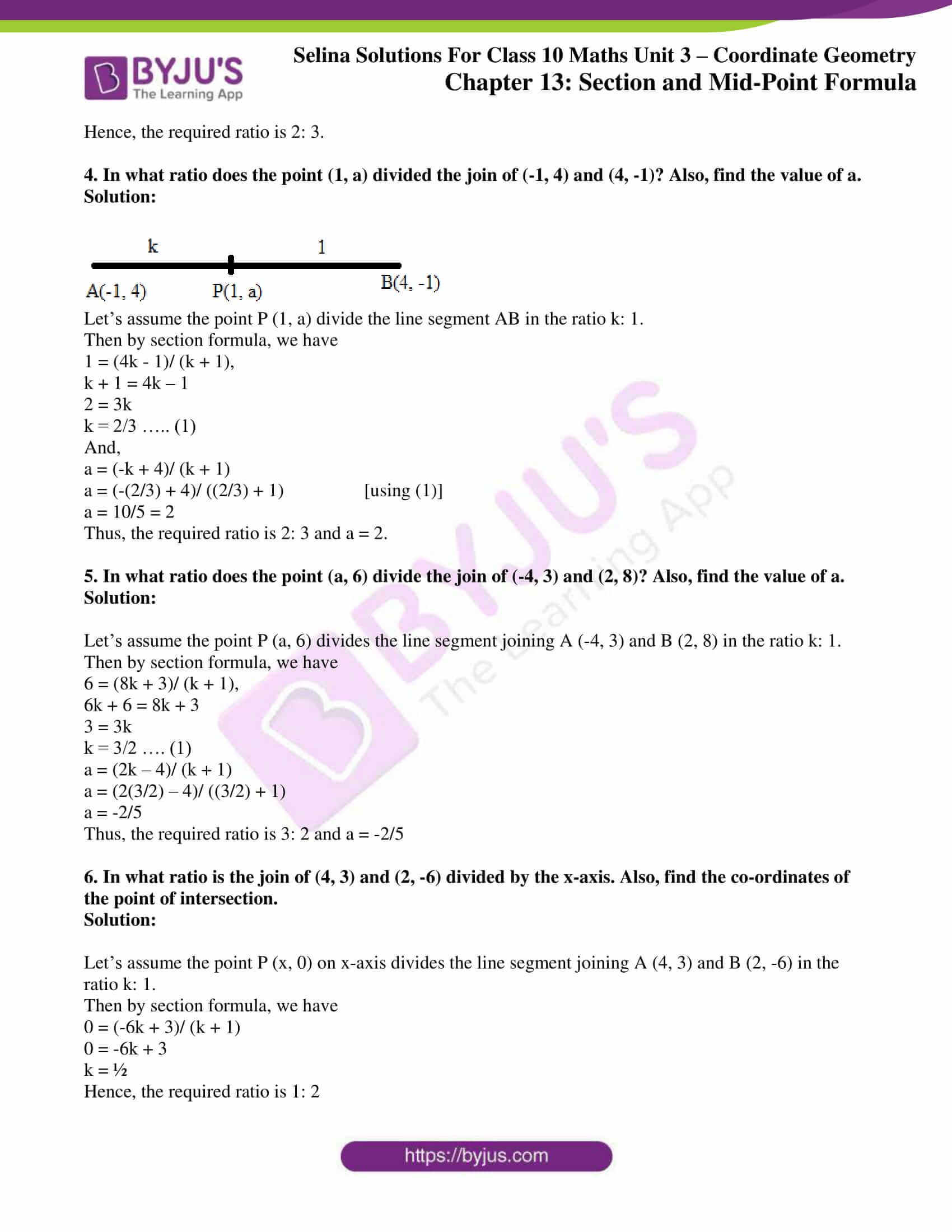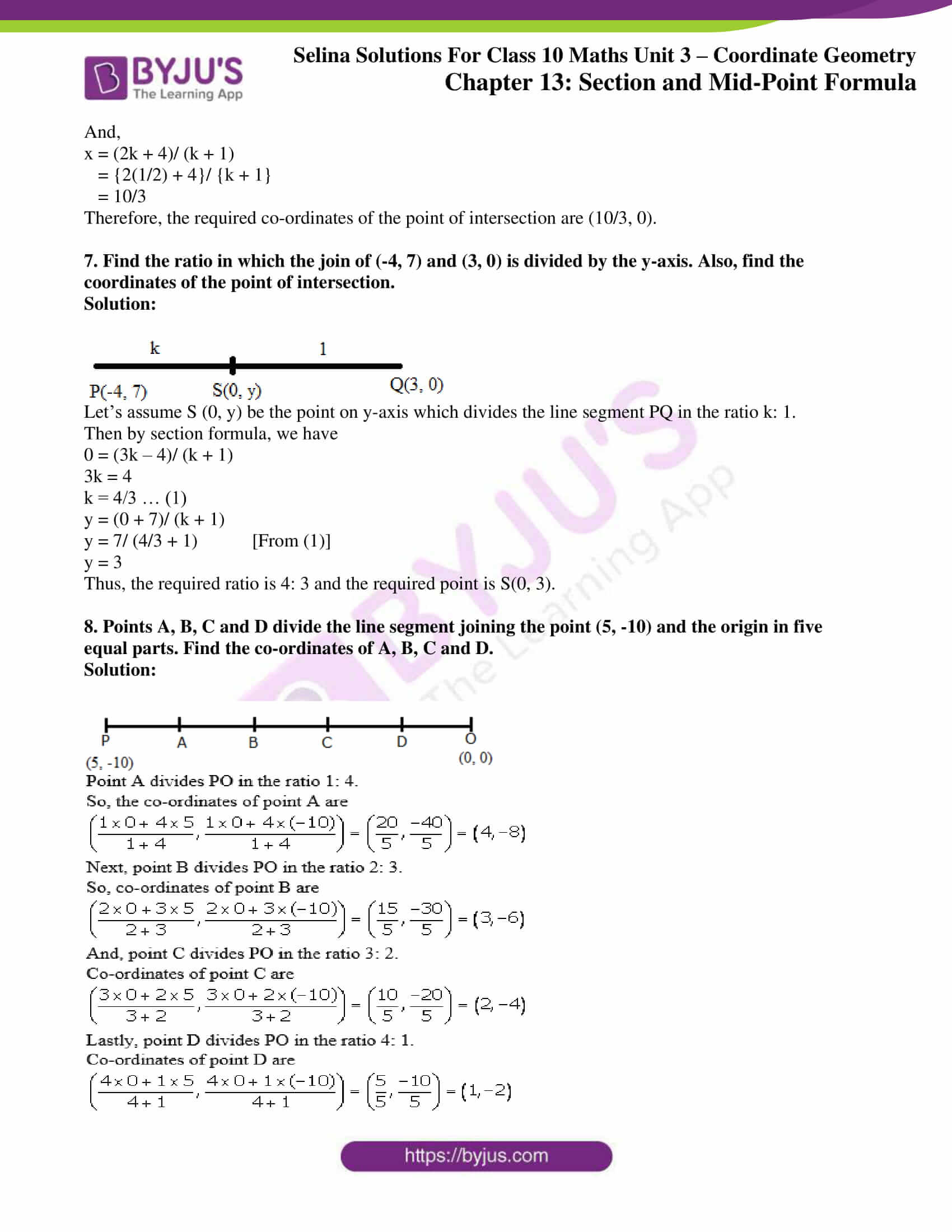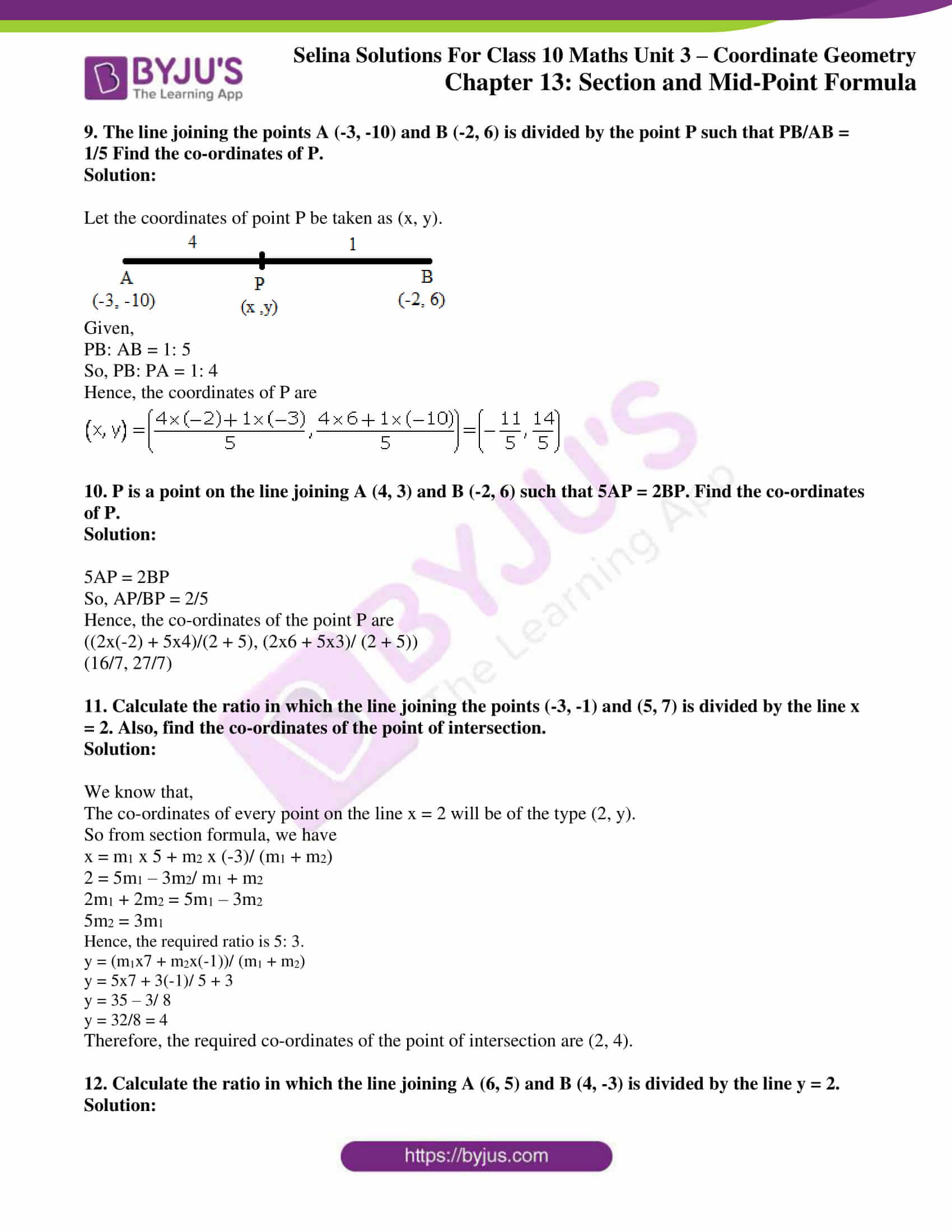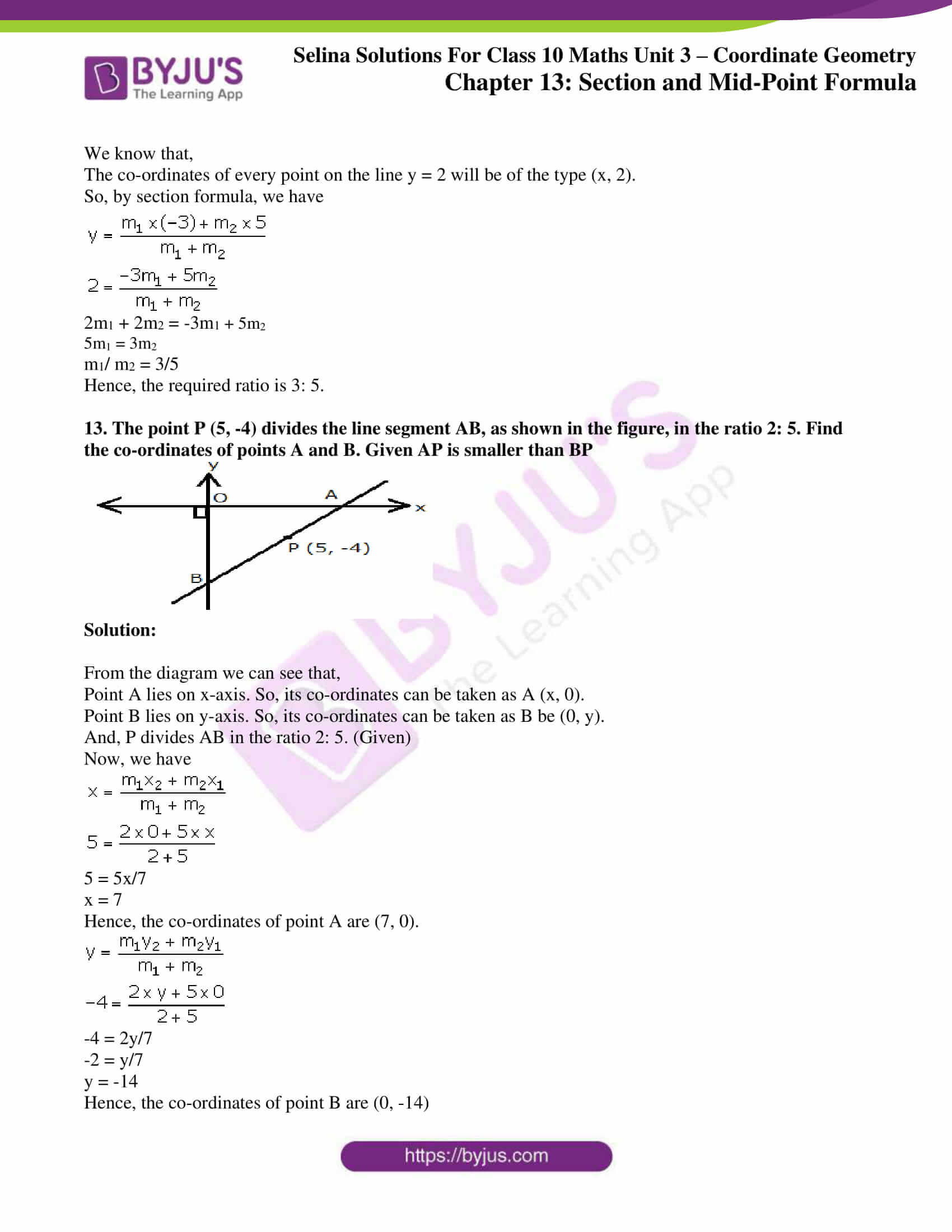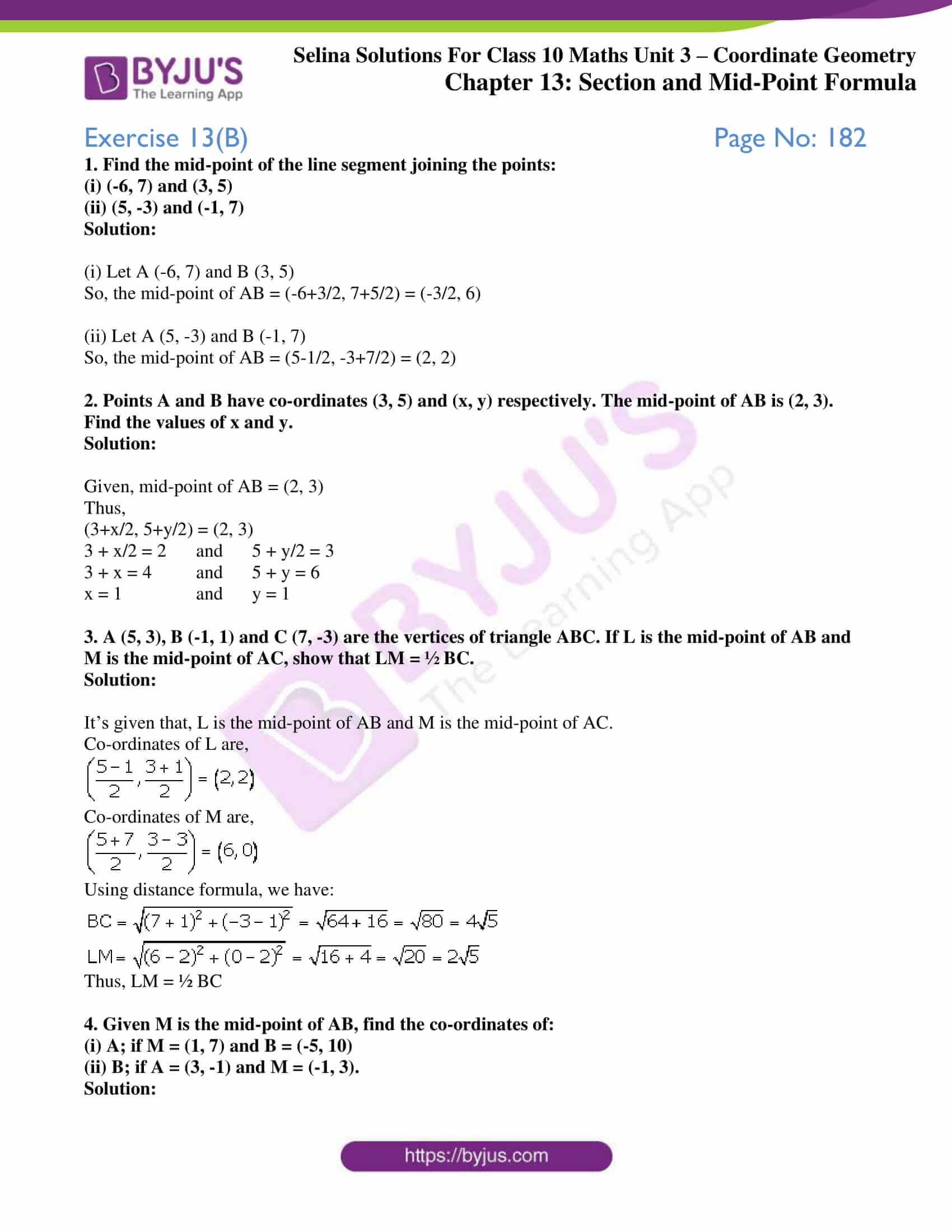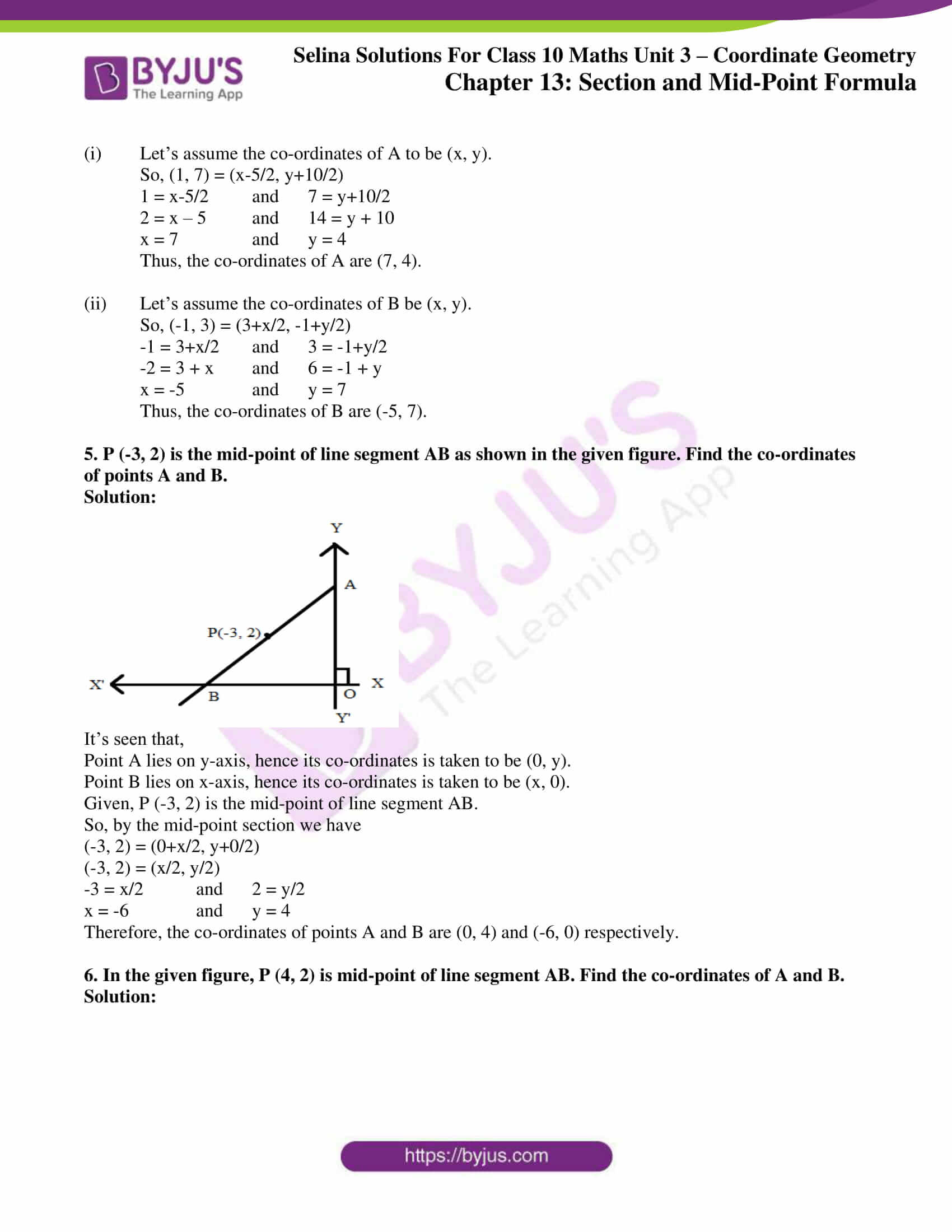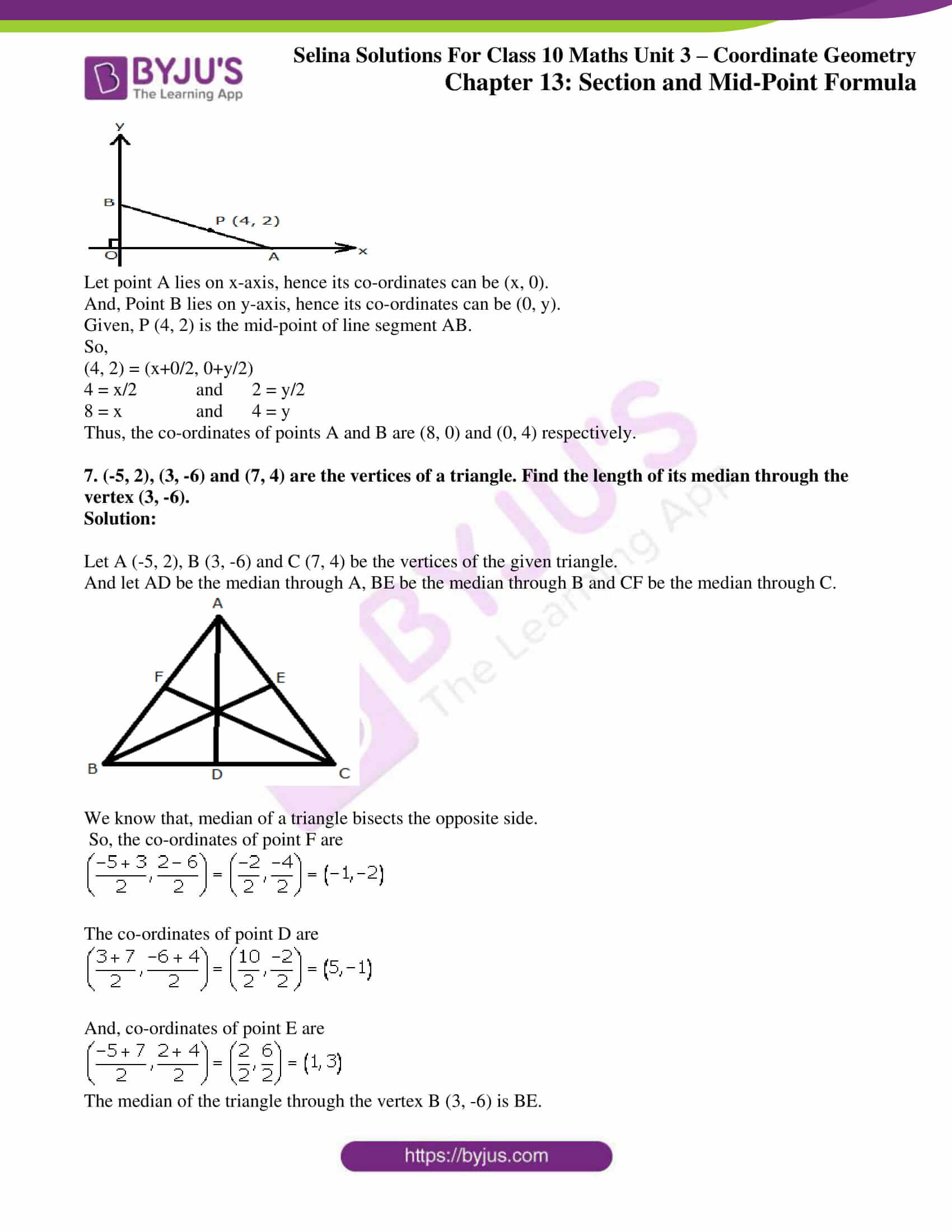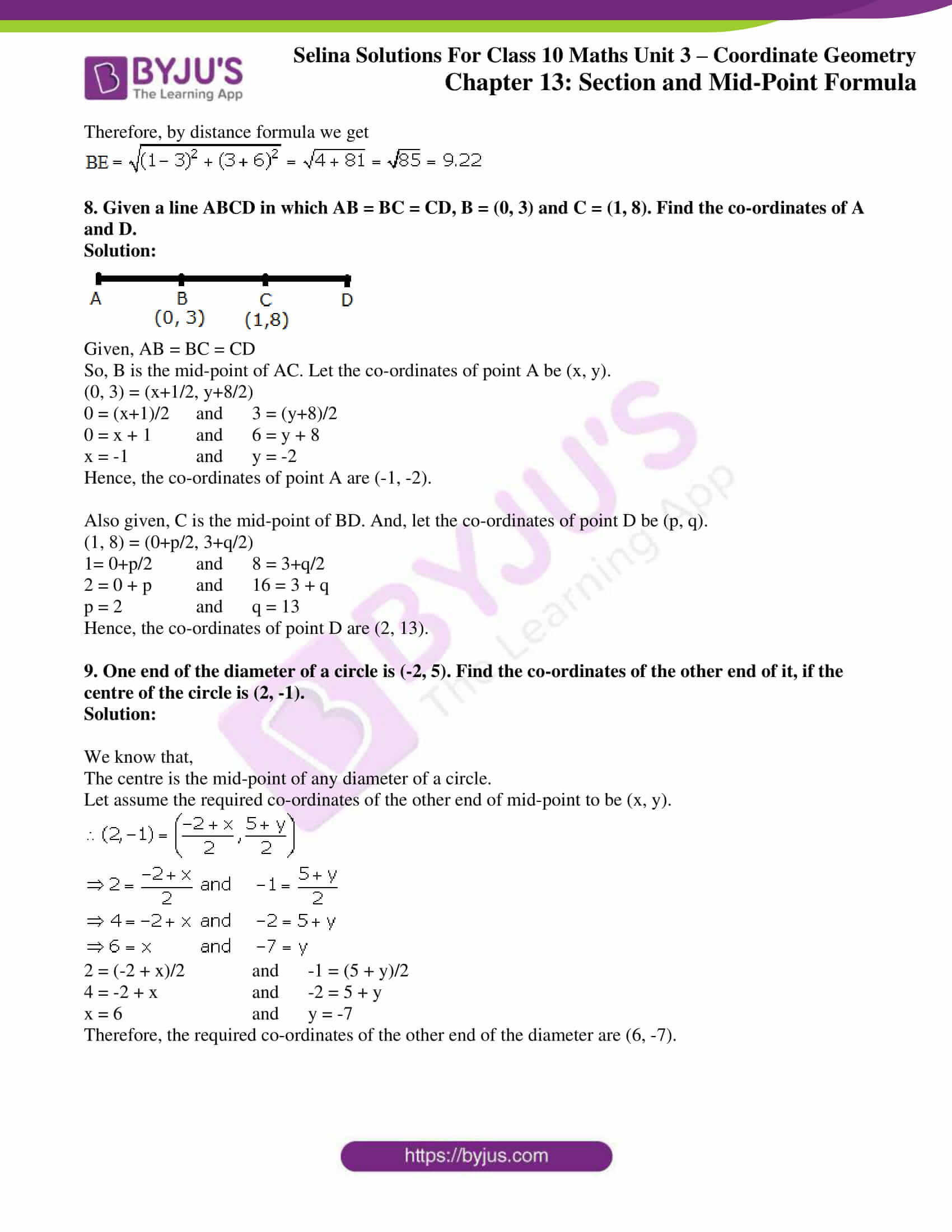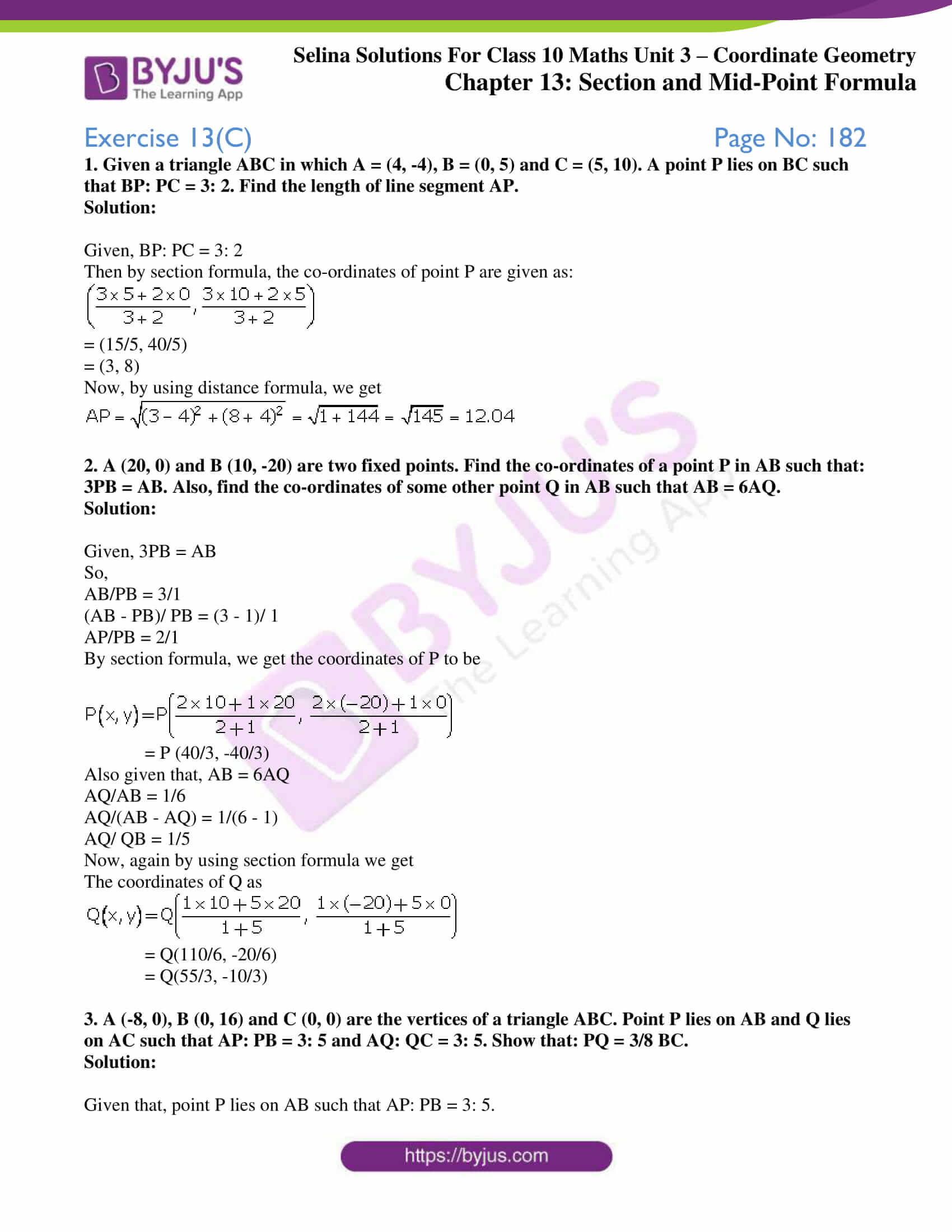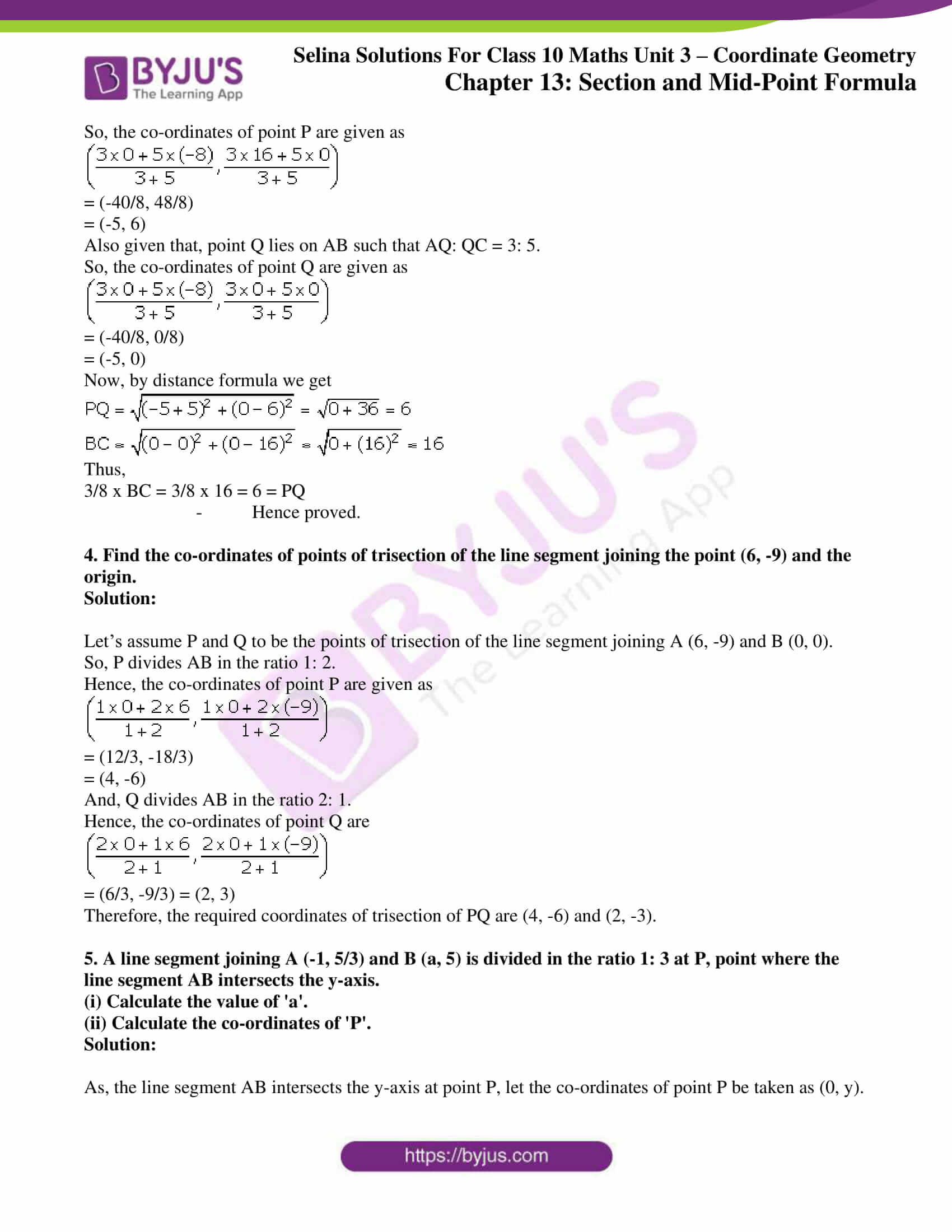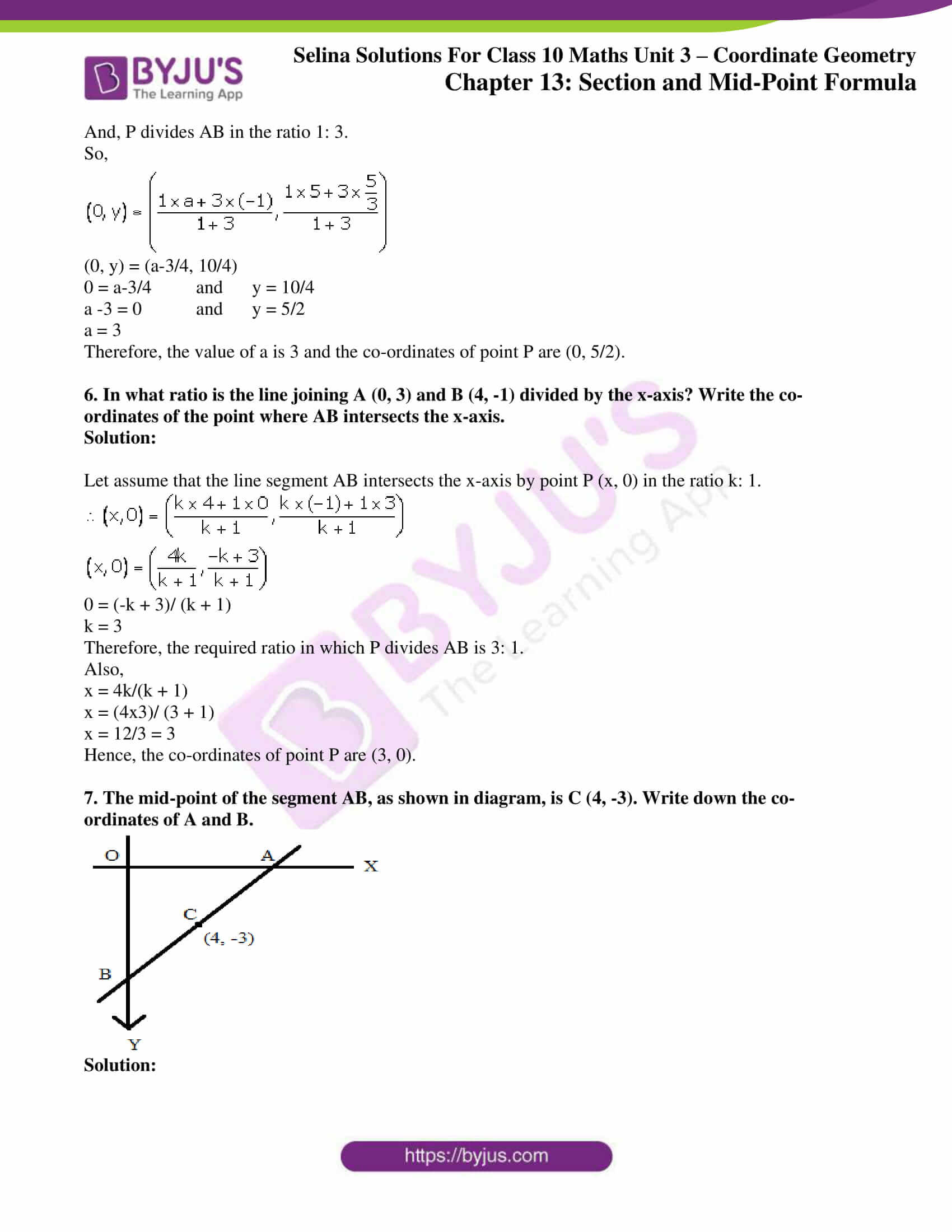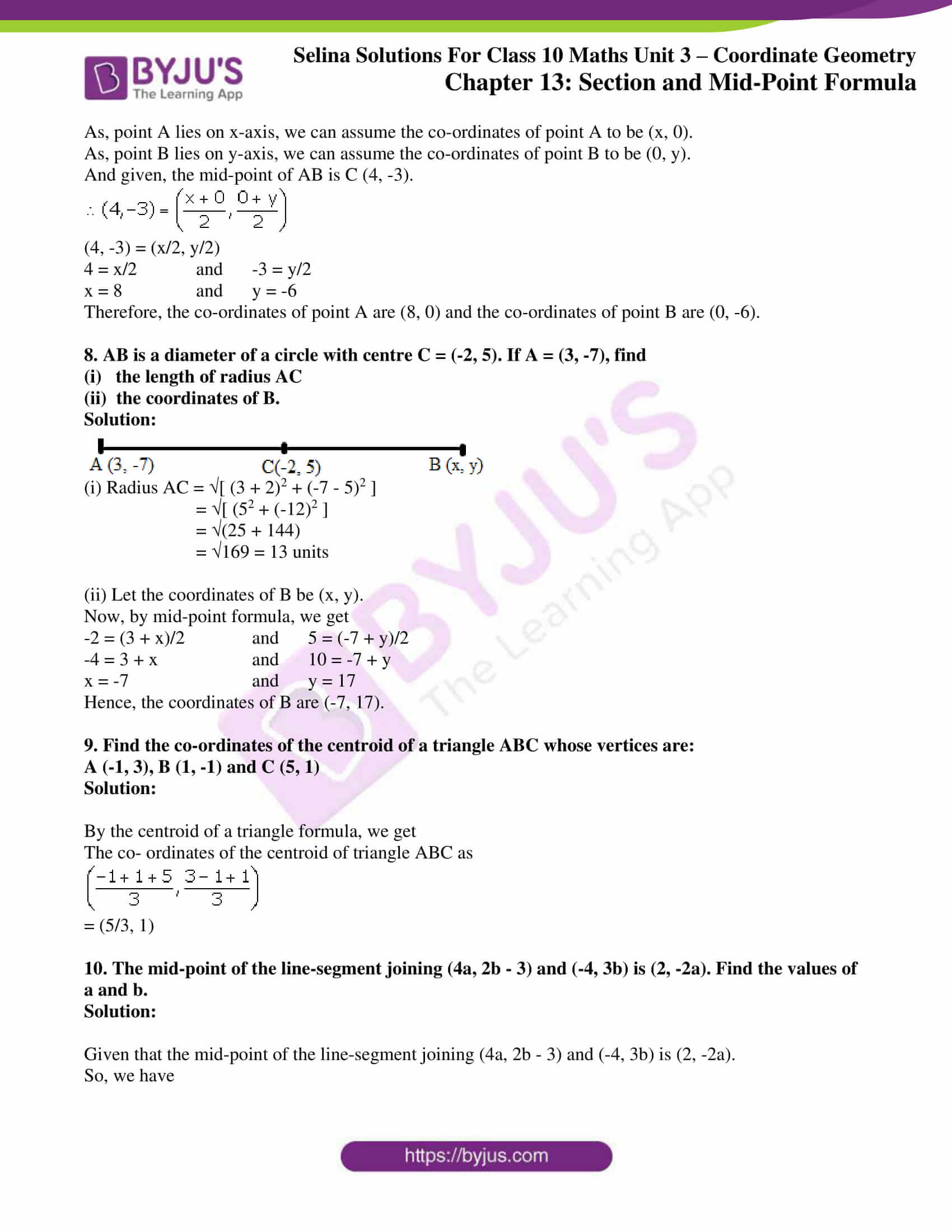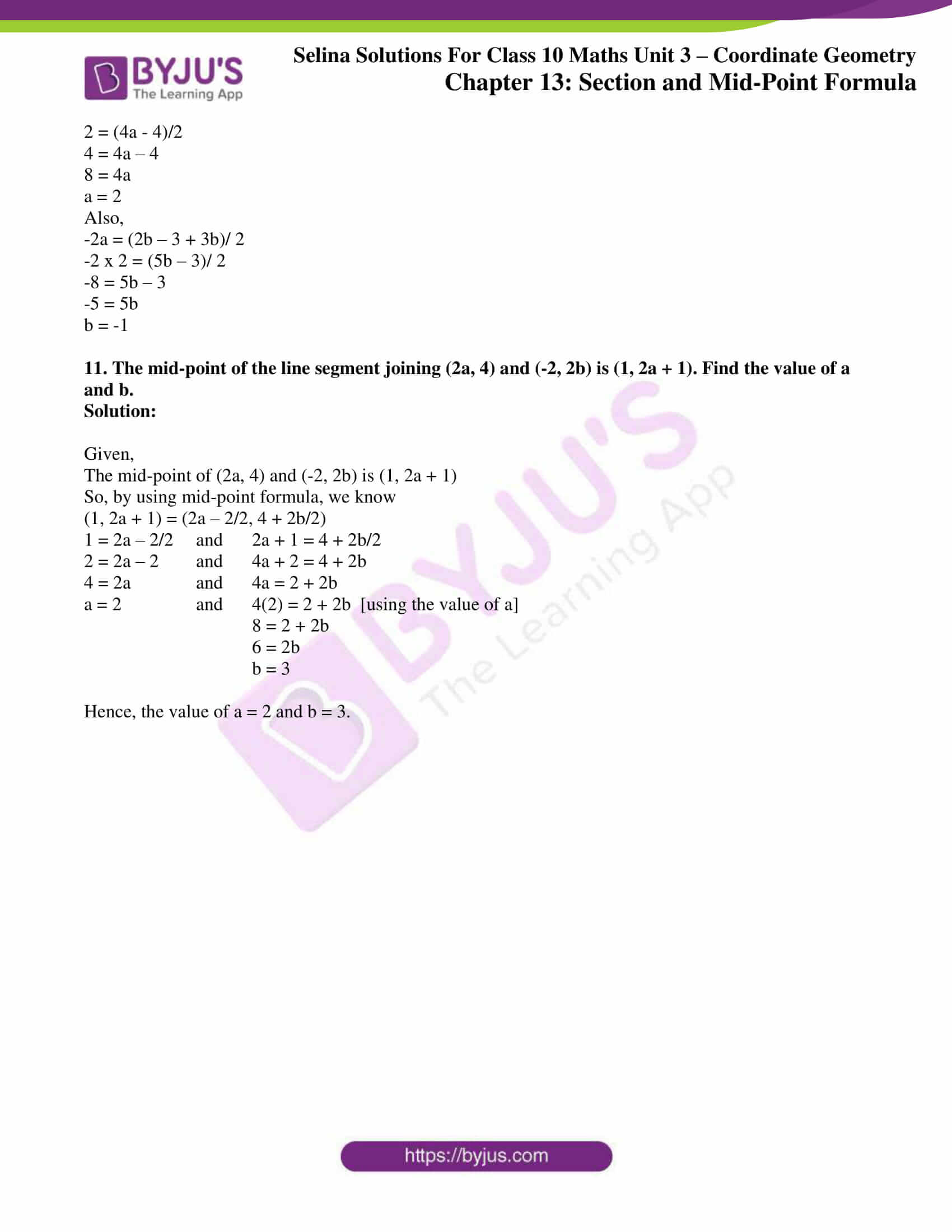### Exercises of Concise Selina Solutions Class 10 Maths Chapter 13 Section and Mid-Point Formula

Exercise 13(A) Solutions

Exercise 13(B) Solutions

Exercise 13(C) Solutions

## Access Selina Solutions Concise Maths Class 10 Chapter 13 Section and Mid-Point Formula

Exercise 13(A) Page No: 177

1. Calculate the co-ordinates of the point P which divides the line segment joining:

(i) A (1, 3) and B (5, 9) in the ratio 1: 2.

(ii) A (-4, 6) and B (3, -5) in the ratio 3: 2.

Solution:

(i) Let’s assume the co-ordinates of the point P be (x, y)

Then by section formula, we have

P(x, y) = (m1x2 + m2x1)/ (m1 + m2), (m1y2 + m2y1)/ (m1 + m2)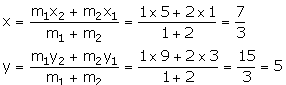Hence, the co-ordinates of point P are (7/3, 5).

(ii) Let’s assume the co-ordinates of the point P be (x, y)

Then by section formula, we have

P(x, y) = (m1x2 + m2x1)/ (m1 + m2), (m1y2 + m2y1)/ (m1 + m2)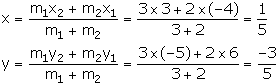Hence, the co-ordinates of point P are (1/5, -3/5).

2. In what ratio is the line joining (2, -3) and (5, 6) divided by the x-axis.

Solution:

Let’s assume the joining points as A(2, -3) and B(5, 6) be divided by point P(x ,0) in the ratio k: 1.

Then we have,

y = ky2 + y1/ (k + 1)

0 = 6k + (-3)/ (k + 1)

0 = 6k – 3

k = ½

Hence, the required ratio is 1: 2.

3. In what ratio is the line joining (2, -4) and (-3, 6) divided by the y-axis.

Solution:

Let’s assume the line joining points A(2, -4) and B(-3, 6) be divided by point P (0, y) in the ratio k: 1.

Then we have,

x = kx2 + x1/ (k + 1)

0 = k(-3) + (1×2)/ (k + 1)

0 = -3k + 2

k = 2/3

Hence, the required ratio is 2: 3.

4. In what ratio does the point (1, a) divided the join of (-1, 4) and (4, -1)? Also, find the value of a.

Solution: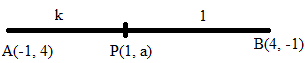Let’s assume the point P (1, a) divide the line segment AB in the ratio k: 1.

Then by section formula, we have

1 = (4k – 1)/ (k + 1),

k + 1 = 4k – 1

2 = 3k

k = 2/3 ….. (1)

And,

a = (-k + 4)/ (k + 1)

a = (-(2/3) + 4)/ ((2/3) + 1) [using (1)]

a = 10/5 = 2

Thus, the required ratio is 2: 3 and a = 2.

5. In what ratio does the point (a, 6) divide the join of (-4, 3) and (2, 8)? Also, find the value of a.

Solution:

Let’s assume the point P (a, 6) divides the line segment joining A (-4, 3) and B (2, 8) in the ratio k: 1.

Then by section formula, we have

6 = (8k + 3)/ (k + 1),

6k + 6 = 8k + 3

3 = 3k

k = 3/2 …. (1)

a = (2k – 4)/ (k + 1)

a = (2(3/2) – 4)/ ((3/2) + 1)

a = -2/5

Thus, the required ratio is 3: 2 and a = -2/5

6. In what ratio is the join of (4, 3) and (2, -6) divided by the x-axis. Also, find the co-ordinates of the point of intersection.

Solution:

Let’s assume the point P (x, 0) on x-axis divides the line segment joining A (4, 3) and B (2, -6) in the ratio k: 1.

Then by section formula, we have

0 = (-6k + 3)/ (k + 1)

0 = -6k + 3

k = ½

Hence, the required ratio is 1: 2

And,

x = (2k + 4)/ (k + 1)

= {2(1/2) + 4}/ {k + 1}

= 10/3

Therefore, the required co-ordinates of the point of intersection are (10/3, 0).

7. Find the ratio in which the join of (-4, 7) and (3, 0) is divided by the y-axis. Also, find the coordinates of the point of intersection.

Solution: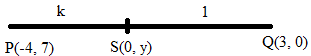Let’s assume S (0, y) be the point on y-axis which divides the line segment PQ in the ratio k: 1.

Then by section formula, we have

0 = (3k – 4)/ (k + 1)

3k = 4

k = 4/3 … (1)

y = (0 + 7)/ (k + 1)

y = 7/ (4/3 + 1) [From (1)]

y = 3

Thus, the required ratio is 4: 3 and the required point is S(0, 3).

8. Points A, B, C and D divide the line segment joining the point (5, -10) and the origin in five equal parts. Find the co-ordinates of A, B, C and D.

Solution: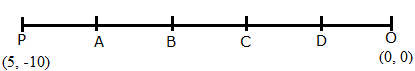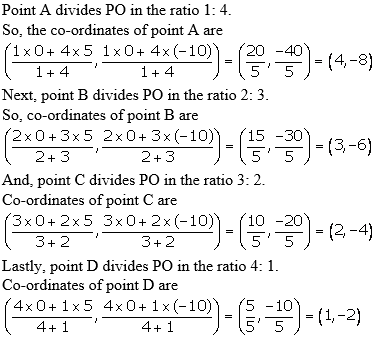9. The line joining the points A (-3, -10) and B (-2, 6) is divided by the point P such that PB/AB = 1/5 Find the co-ordinates of P.

Solution:

Let the coordinates of point P be taken as (x, y).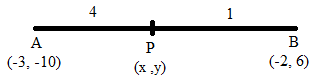Given,

PB: AB = 1: 5

So, PB: PA = 1: 4

Hence, the coordinates of P are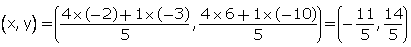10. P is a point on the line joining A (4, 3) and B (-2, 6) such that 5AP = 2BP. Find the co-ordinates of P.

Solution:

5AP = 2BP

So, AP/BP = 2/5

Hence, the co-ordinates of the point P are

((2x(-2) + 5×4)/(2 + 5), (2×6 + 5×3)/ (2 + 5))

(16/7, 27/7)

11. Calculate the ratio in which the line joining the points (-3, -1) and (5, 7) is divided by the line x = 2. Also, find the co-ordinates of the point of intersection.

Solution:

We know that,

The co-ordinates of every point on the line x = 2 will be of the type (2, y).

So from section formula, we have

x = m1 x 5 + m2 x (-3)/ (m1 + m2)

2 = 5m1 – 3m2/ m1 + m2

2m1 + 2m2 = 5m1 – 3m2

5m2 = 3m1

Hence, the required ratio is 5: 3.

y = (m1x7 + m2x(-1))/ (m1 + m2)

y = 5×7 + 3(-1)/ 5 + 3

y = 35 – 3/ 8

y = 32/8 = 4

Therefore, the required co-ordinates of the point of intersection are (2, 4).

12. Calculate the ratio in which the line joining A (6, 5) and B (4, -3) is divided by the line y = 2.

Solution:

We know that,

The co-ordinates of every point on the line y = 2 will be of the type (x, 2).

So, by section formula, we have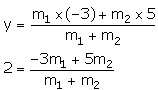2m1 + 2m2 = -3m1 + 5m2

5m1 = 3m2

m1/ m2 = 3/5

Hence, the required ratio is 3: 5.

13. The point P (5, -4) divides the line segment AB, as shown in the figure, in the ratio 2: 5. Find the co-ordinates of points A and B. Given AP is smaller than BP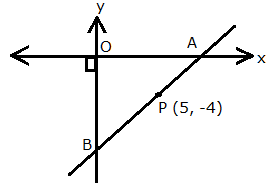Solution:

From the diagram we can see that,

Point A lies on x-axis. So, its co-ordinates can be taken as A (x, 0).

Point B lies on y-axis. So, its co-ordinates can be taken as B be (0, y).

And, P divides AB in the ratio 2: 5. (Given)

Now, we have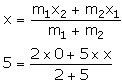5 = 5x/7

x = 7

Hence, the co-ordinates of point A are (7, 0).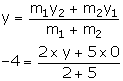-4 = 2y/7

-2 = y/7

y = -14

Hence, the co-ordinates of point B are (0, -14)

Exercise 13(B) Page No: 182

1. Find the mid-point of the line segment joining the points:

(i) (-6, 7) and (3, 5)

(ii) (5, -3) and (-1, 7)

Solution:

(i) Let A (-6, 7) and B (3, 5)

So, the mid-point of AB = (-6+3/2, 7+5/2) = (-3/2, 6)

(ii) Let A (5, -3) and B (-1, 7)

So, the mid-point of AB = (5-1/2, -3+7/2) = (2, 2)

2. Points A and B have co-ordinates (3, 5) and (x, y) respectively. The mid-point of AB is (2, 3). Find the values of x and y.

Solution:

Given, mid-point of AB = (2, 3)

Thus,

(3+x/2, 5+y/2) = (2, 3)

3 + x/2 = 2 and 5 + y/2 = 3

3 + x = 4 and 5 + y = 6

x = 1 and y = 1

3. A (5, 3), B (-1, 1) and C (7, -3) are the vertices of triangle ABC. If L is the mid-point of AB and M is the mid-point of AC, show that LM = ½ BC.

Solution:

It’s given that, L is the mid-point of AB and M is the mid-point of AC.

Co-ordinates of L are,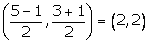Co-ordinates of M are,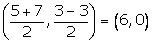Using distance formula, we have: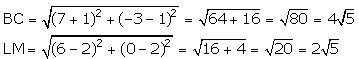Thus, LM = ½ BC

4. Given M is the mid-point of AB, find the co-ordinates of:

(i) A; if M = (1, 7) and B = (-5, 10)

(ii) B; if A = (3, -1) and M = (-1, 3).

Solution:

(i) Let’s assume the co-ordinates of A to be (x, y).

So, (1, 7) = (x-5/2, y+10/2)

1 = x-5/2 and 7 = y+10/2

2 = x – 5 and 14 = y + 10

x = 7 and y = 4

Thus, the co-ordinates of A are (7, 4).

(ii) Let’s assume the co-ordinates of B be (x, y).

So, (-1, 3) = (3+x/2, -1+y/2)

-1 = 3+x/2 and 3 = -1+y/2

-2 = 3 + x and 6 = -1 + y

x = -5 and y = 7

Thus, the co-ordinates of B are (-5, 7).

5. P (-3, 2) is the mid-point of line segment AB as shown in the given figure. Find the co-ordinates of points A and B.

Solution: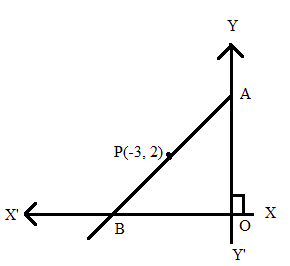It’s seen that,

Point A lies on y-axis, hence its co-ordinates is taken to be (0, y).

Point B lies on x-axis, hence its co-ordinates is taken to be (x, 0).

Given, P (-3, 2) is the mid-point of line segment AB.

So, by the mid-point section we have

(-3, 2) = (0+x/2, y+0/2)

(-3, 2) = (x/2, y/2)

-3 = x/2 and 2 = y/2

x = -6 and y = 4

Therefore, the co-ordinates of points A and B are (0, 4) and (-6, 0) respectively.

6. In the given figure, P (4, 2) is mid-point of line segment AB. Find the co-ordinates of A and B.

Solution: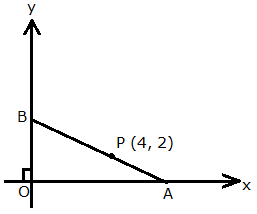Let point A lies on x-axis, hence its co-ordinates can be (x, 0).

And, Point B lies on y-axis, hence its co-ordinates can be (0, y).

Given, P (4, 2) is the mid-point of line segment AB.

So,

(4, 2) = (x+0/2, 0+y/2)

4 = x/2 and 2 = y/2

8 = x and 4 = y

Thus, the co-ordinates of points A and B are (8, 0) and (0, 4) respectively.

7. (-5, 2), (3, -6) and (7, 4) are the vertices of a triangle. Find the length of its median through the vertex (3, -6).
Solution:

Let A (-5, 2), B (3, -6) and C (7, 4) be the vertices of the given triangle.

And let AD be the median through A, BE be the median through B and CF be the median through C.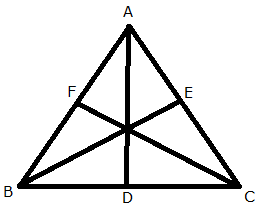We know that, median of a triangle bisects the opposite side.

So, the co-ordinates of point F are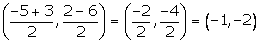The co-ordinates of point D are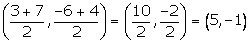And, co-ordinates of point E are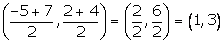The median of the triangle through the vertex B (3, -6) is BE.

Therefore, by distance formula we get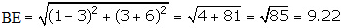8. Given a line ABCD in which AB = BC = CD, B = (0, 3) and C = (1, 8). Find the co-ordinates of A and D.

Solution: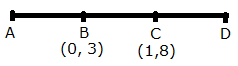Given, AB = BC = CD

So, B is the mid-point of AC. Let the co-ordinates of point A be (x, y).

(0, 3) = (x+1/2, y+8/2)

0 = (x+1)/2 and 3 = (y+8)/2

0 = x + 1 and 6 = y + 8

x = -1 and y = -2

Hence, the co-ordinates of point A are (-1, -2).

Also given, C is the mid-point of BD. And, let the co-ordinates of point D be (p, q).

(1, 8) = (0+p/2, 3+q/2)

1= 0+p/2 and 8 = 3+q/2

2 = 0 + p and 16 = 3 + q

p = 2 and q = 13

Hence, the co-ordinates of point D are (2, 13).

9. One end of the diameter of a circle is (-2, 5). Find the co-ordinates of the other end of it, if the centre of the circle is (2, -1).

Solution:

We know that,

The centre is the mid-point of any diameter of a circle.

Let assume the required co-ordinates of the other end of mid-point to be (x, y).2 = (-2 + x)/2 and -1 = (5 + y)/2

4 = -2 + x and -2 = 5 + y

x = 6 and y = -7

Therefore, the required co-ordinates of the other end of the diameter are (6, -7).

Exercise 13(C) Page No: 182

1. Given a triangle ABC in which A = (4, -4), B = (0, 5) and C = (5, 10). A point P lies on BC such that BP: PC = 3: 2. Find the length of line segment AP.

Solution:

Given, BP: PC = 3: 2

Then by section formula, the co-ordinates of point P are given as:= (15/5, 40/5)

= (3, 8)

Now, by using distance formula, we get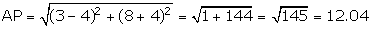2. A (20, 0) and B (10, -20) are two fixed points. Find the co-ordinates of a point P in AB such that: 3PB = AB. Also, find the co-ordinates of some other point Q in AB such that AB = 6AQ.

Solution:

Given, 3PB = AB

So,

AB/PB = 3/1

(AB – PB)/ PB = (3 – 1)/ 1

AP/PB = 2/1

By section formula, we get the coordinates of P to be= P (40/3, -40/3)

Also given that, AB = 6AQ

AQ/AB = 1/6

AQ/(AB – AQ) = 1/(6 – 1)

AQ/ QB = 1/5

Now, again by using section formula we get

The coordinates of Q as= Q(110/6, -20/6)

= Q(55/3, -10/3)

3. A (-8, 0), B (0, 16) and C (0, 0) are the vertices of a triangle ABC. Point P lies on AB and Q lies on AC such that AP: PB = 3: 5 and AQ: QC = 3: 5. Show that: PQ = 3/8 BC.

Solution:

Given that, point P lies on AB such that AP: PB = 3: 5.

So, the co-ordinates of point P are given as= (-40/8, 48/8)

= (-5, 6)

Also given that, point Q lies on AB such that AQ: QC = 3: 5.

So, the co-ordinates of point Q are given as= (-40/8, 0/8)

= (-5, 0)

Now, by distance formula we getThus,

3/8 x BC = 3/8 x 16 = 6 = PQ

– Hence proved.

4. Find the co-ordinates of points of trisection of the line segment joining the point (6, -9) and the origin.

Solution:

Let’s assume P and Q to be the points of trisection of the line segment joining A (6, -9) and B (0, 0).

So, P divides AB in the ratio 1: 2.

Hence, the co-ordinates of point P are given as= (12/3, -18/3)

= (4, -6)

And, Q divides AB in the ratio 2: 1.

Hence, the co-ordinates of point Q are= (6/3, -9/3) = (2, 3)

Therefore, the required coordinates of trisection of PQ are (4, -6) and (2, -3).

5. A line segment joining A (-1, 5/3) and B (a, 5) is divided in the ratio 1: 3 at P, point where the line segment AB intersects the y-axis.

(i) Calculate the value of ‘a’.

(ii) Calculate the co-ordinates of ‘P’.

Solution:

As, the line segment AB intersects the y-axis at point P, let the co-ordinates of point P be taken as (0, y).

And, P divides AB in the ratio 1: 3.

So,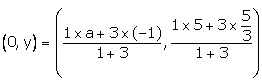(0, y) = (a-3/4, 10/4)

0 = a-3/4 and y = 10/4

a -3 = 0 and y = 5/2

a = 3

Therefore, the value of a is 3 and the co-ordinates of point P are (0, 5/2).

6. In what ratio is the line joining A (0, 3) and B (4, -1) divided by the x-axis? Write the co-ordinates of the point where AB intersects the x-axis.

Solution:

Let assume that the line segment AB intersects the x-axis by point P (x, 0) in the ratio k: 1.0 = (-k + 3)/ (k + 1)

k = 3

Therefore, the required ratio in which P divides AB is 3: 1.

Also,

x = 4k/(k + 1)

x = (4×3)/ (3 + 1)

x = 12/3 = 3

Hence, the co-ordinates of point P are (3, 0).

7. The mid-point of the segment AB, as shown in diagram, is C (4, -3). Write down the co-ordinates of A and B.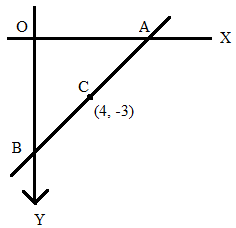Solution:

As, point A lies on x-axis, we can assume the co-ordinates of point A to be (x, 0).

As, point B lies on y-axis, we can assume the co-ordinates of point B to be (0, y).

And given, the mid-point of AB is C (4, -3).(4, -3) = (x/2, y/2)

4 = x/2 and -3 = y/2

x = 8 and y = -6

Therefore, the co-ordinates of point A are (8, 0) and the co-ordinates of point B are (0, -6).

8. AB is a diameter of a circle with centre C = (-2, 5). If A = (3, -7), find

(i)   the length of radius AC

(ii)  the coordinates of B.

Solution: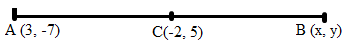(i) Radius AC = √[ (3 + 2)2 + (-7 – 5)2 ]

= √[ (52 + (-12)2 ]

= √(25 + 144)

= √169 = 13 units

(ii) Let the coordinates of B be (x, y).

Now, by mid-point formula, we get

-2 = (3 + x)/2 and 5 = (-7 + y)/2

-4 = 3 + x and 10 = -7 + y

x = -7 and y = 17

Hence, the coordinates of B are (-7, 17).

9. Find the co-ordinates of the centroid of a triangle ABC whose vertices are:

A (-1, 3), B (1, -1) and C (5, 1)

Solution:

By the centroid of a triangle formula, we get

The co- ordinates of the centroid of triangle ABC as= (5/3, 1)

10. The mid-point of the line-segment joining (4a, 2b – 3) and (-4, 3b) is (2, -2a). Find the values of a and b.

Solution:

Given that the mid-point of the line-segment joining (4a, 2b – 3) and (-4, 3b) is (2, -2a).

So, we have

2 = (4a – 4)/2

4 = 4a – 4

8 = 4a

a = 2

Also,

-2a = (2b – 3 + 3b)/ 2

-2 x 2 = (5b – 3)/ 2

-8 = 5b – 3

-5 = 5b

b = -1

11. The mid-point of the line segment joining (2a, 4) and (-2, 2b) is (1, 2a + 1). Find the value of a and b.

Solution:

Given,

The mid-point of (2a, 4) and (-2, 2b) is (1, 2a + 1)

So, by using mid-point formula, we know

(1, 2a + 1) = (2a – 2/2, 4 + 2b/2)

1 = 2a – 2/2 and 2a + 1 = 4 + 2b/2

2 = 2a – 2 and 4a + 2 = 4 + 2b

4 = 2a and 4a = 2 + 2b

a = 2 and 4(2) = 2 + 2b [using the value of a]

8 = 2 + 2b

6 = 2b

b = 3

Hence, the value of a = 2 and b = 3.

The given solutions are as per the 2019-20 Concise Selina textbook. The Selina Solutions for the academic year 2020-21 will be updated soon.